#### Content of Theoretical Model and GIS Application in our journal

 Published in last 1 year | In last 2 years | In last 3 years | All
 For Selected: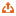Download Citations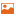Toggle Thumbnails
 Select Simplicity, complexity, and mathematical modeling of geographical distributions Yanguang CHEN PROGRESS IN GEOGRAPHY    2015, 34 (3): 321-329.   DOI: 10.11820/dlkxjz.2015.03.007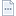Abstract （1229）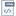HTML （4）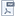PDF （611KB）（2354）       Because of quantitative revolution, geography evolved from a discipline of spatial description into a science of distributions. Accordingly, qualitative methods were replaced by the integrated methods of quantitative analysis and qualitative analysis. One of the important approaches to spatial analysis is to characterize geographical distributions. Geographical distributions fall into spatial distributions and size distributions, both of which can be divided into simple distributions and complex distributions. A simple distribution has a characteristic scale (represented by a characteristic length), while a complex distribution has no characteristic scale but bears a property of scaling invariance (represented by a scaling exponent). The key step of studying a simple distribution is to find its characteristic scale, while the basic way of research for a complex distribution is to make a scaling analysis. For simple distributions, traditional methods based on advanced mathematics are effective, but for complex distributions, the old-fashioned mathematical tools are ineffective. However, due to the lack of understanding of characteristic scale and scaling, geographers often failed to distinguish between simple distributions and complex distributions. As a result, many complex systems such as cities and systems of cities were mistaken for simple systems. Consequently, geography did not succeed in theorization in the 1960s-1970s after quantification in the 1950s-1960s. Geographical distributions can be mathematically abstracted as probability distributions. For a simple distribution, its characteristic scale can be determined. A typical characteristic scale is a mean (average value). Based on means, we can compute a variance and a covariance. Thus we have a clear probability structure comprising means, variances, and covariances, which explain the pattern of the geographical system and predict its process of evolution. In the case of a complex distribution, an effective mean cannot be determined, and thus little is known about its probability structure. In this situation, characteristic scale analysis should be substituted with scaling analysis. Quantitative methods of scaling analysis have emerged from interdisciplinary studies. A new integrated theory based on concepts from fractals, allometry, and complex network has been developing for geographical modeling and analysis.